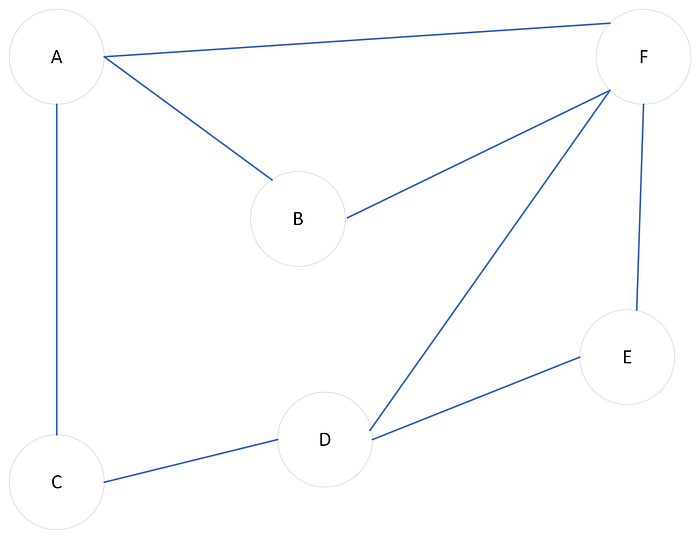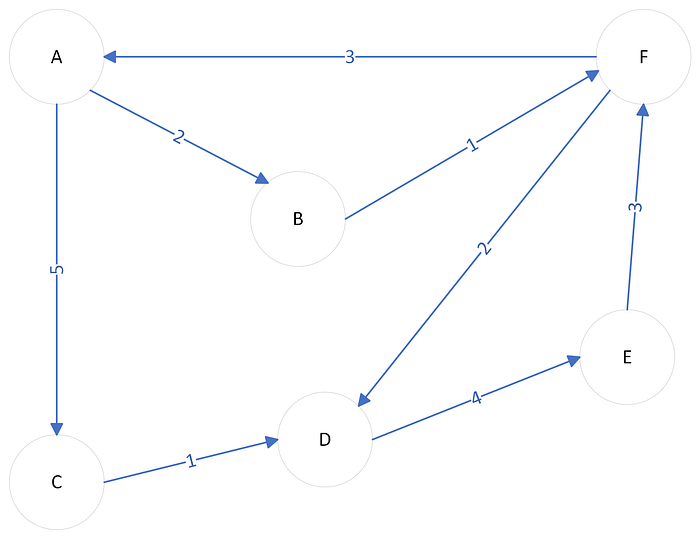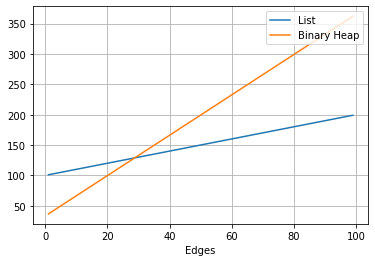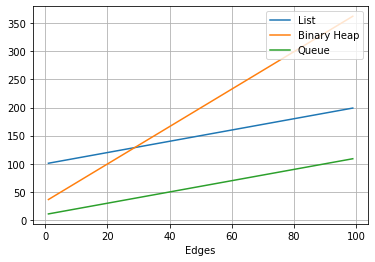# Algorithms and How to Choose the Right Data Structure

Go to the source link to view the article. You can view the picture content, updated content and better typesetting reading experience. If the link is broken, please click the button below to view the snapshot at that time.

# Algorithms and How to Choose the Right Data StructurePhoto by Christina @ wocintechchat.com on Unsplash

## You cannot talk about algorithms without mentioning the importance of data structures.

Different data structure choices can have an impact on the performance of an algorithm. Depending on your choice, the right pick can lead to more efficiency. Furthermore, Data Structures are one of the foundational topics in Computer Science, it is important to consolidate a solid knowledge regarding this subject and how it can be applied in your everyday life as a Software Engineer.

In this story, we are going to focus on how data structures affect algorithms in terms of execution performance and some guidelines on how to choose it.

# The Purpose of Data Structures

They serve as abstractions that allow us to organize data to keep, retrieve and use whenever they are needed. A data structure helps us to treat such data logically. Data Structures define how data is stored and accessed in memory and how it can be processed.

Running an algorithm involves storing and manipulating data, this will require a certain number of operations to be done using it.

Some of the most common operations include:

• Search
• Access
• Insert
• Delete

In each data structure, such operations will be performed at a certain cost, which is commonly expressed in terms of the big-O notation.

You can refer to this article from Free Code Camp for more details about the notation itself. In summary, it is a convention for measuring the performance of algorithms or operations as a function of their inputs.

There are basic types of data structures that are commonly available in many programming languages such as Integers, Float Numbers, Strings, and Boolean.

They are the building blocks of more complex structures such as Queue, Stack, Array, Linked-List, and Hash Table.

For details about the complexity costs involving common data structures, you can refer to the Big-O Cheat Sheet that is available here.

# How to choose the right data structure

It is important to state that no single data structure serves as an optimal solution for all problems. With that in mind, to pick the best data structure to solve a particular problem, we have to take into account what are the operations we are going to perform and how often they are going to be executed. Then, we need to choose the data structure that delivers the best performance trade-off over the tasks we have to accomplish.

Let us try to grasp this concept through a concrete example.

## The Shortest Path Algorithm

Imagine you are searching on Google Maps for the best way of getting to a local restaurant from your home.

First of all, we need a way of representing the city map so that we can treat it in our algorithm. One of the possible ways is to represent it as a graph.

## Graph Representation

A graph is a structure composed of nodes(also referred to as vertices) related to each other through edges(links).Graph example. Image by Author

We can define a path as a sequence of consecutive arcs linking one node to the other. Also, the crossing of each edge normally requires a certain cost, in the example of the shortest path algorithm, we can think of it as a distance representation.

This was the example of a graph without directions. We can also have directed graphs, where the relation between nodes would be represented by arrows instead of simple lines.Weighted graph. Image by Author

For instance, the distance between the nodes C and E would be:

`C -> D(Cost 1) + D -> E(Cost 4)Cost 5 in total.`

## Dijkstra’s Algorithms

Now that we have an abstraction of the map. We can select an algorithm to solve the minimum distance problem. In this example, we are going to see the impact of the data structure choice over Dijkstra’s Algorithms.

In a Nutshell, it performs a layer-by-layer traversal of the graph starting at an origin node. As it continues, it keeps a record containing the shortest paths encountered so far for each node found along the way. The shortest path record gets updated whenever we find a better alternative to an existing path.

Algorithm:

1. Declare a list of the nodes to be visited(commonly known as a frontier) starting with the origin node in it.
2. We must assign a distance to every node in the graph from the origin, as we are just starting the algorithm, we can consider such distances as infinite.
3. Start iterating over the list of nodes to be visited:

a. Extract the first node from the frontier

b. Get the node neighbors.

c. Append the neighbors that have not been visited at the end of the frontier list.

d. Compute the distance between the departure node and the node being visited at the current iteration.

e. If the computed distance is smaller than the one already found, update it.

For the frontier, we need a data structure that allows us to easily append data to the end of it and remove its first element. We are going to compare the performances of three data structures:

• Array
• Binary Heap
• Queue

## Array Implementation

In a first trial, we are going to use an array, represented as a Python List, it has the following operation performances:

`Append: O(1)Extract 1st element: O(n)`

Here is the implementation in Python:

We are representing the graph input as a Python Dictionary(Hash Table), where each key corresponds to a node, and each value to another dictionary with the node’s neighbors:

`{ 'A': {'B': 2, 'C': 5}, 'B': {'F': 1}, 'C': {'D': 1}, 'D': {'E': 4}, 'E': {'F': 3}, 'F': {'A': 3, 'D': 2},}`

Analyzing the time complexity of the implementation above, we can identify most notably the following steps:

1. Algorithm init done at constant time
2. While loop that iterates over all the nodes of the graph: O(Nodes)
3. Get the 1st element of the list: O(Extraction).
4. For-loop that iterates over all the edges of the graph: O(Edges) in total.
5. Append complexity: O(Append).

Overall, our implementation yields the following complexity:

`O(Nodes.Extraction + Edges.Append)`

Using the Python List, we have:

`O(Extraction) = O(Nodes) at worstO(Append) = O(1)`

The final complexity will be:

`O(Nodes² + Edges)`

## Binary Heap Implementation

Notice that the choice of the Array data structure has a certain impact on the performance, knowing that the operation to extract the 1st element comes at the cost of:

`O(Nodes)`

The Binary Heap apparently would deliver a better complexity trade-off:

`Append: O(log n)Extract 1st element: O(log n)`

We can change the underlying frontier implementation by altering the Append and Extract functions:

The new complexity corresponds to:

`O(log Nodes.(Nodes + Edges))`

## Is it actually better?

It depends on the number of edges we have on the graph, for a fixed number of 10 Nodes:Heap Comparison. Image by Author

The performance using the binary heap is better only for a number of Edges below 30.

## Queue Implementation

We can still try to improve this performance using an alternative data structure, the Queue can be a better choice in this case since it has:

`Append: O(1)Extract: O(1)`

Both operations can be done at constant time! This is surely going to improve our performance:

This choice will lead to the following complexity:

`O(Nodes + Edges)`Queue Comparison. Image by Author

The image above shows that the queue yields the best performance for the shortest distance algorithm. No matter the number of edges of the graph.

# Conclusion

In this article, we have seen the impact the data structure has on the execution of an algorithm, here are the key takeaways:

• No single data structure can be used as a solution to every problem.
• To make the best choice possible, we have to consider how the data structure will be used and how often.
• Try to choose the one that has the lowest costs for the operations done the most often.
• Compare different approaches! Maybe it is not that obvious to find which one will suit the best.

Thanks for making it to the end! If these insights have been helpful, consider following me on Medium for more stories like this one!

# Bit: Build Better UI Component Libraries

Say hey to Bit. It’s the #1 tool for component-driven app development.

With Bit, you can create any part of your app as a “component” that’s composable and reusable. You and your team can share a toolbox of components to build more apps faster and consistently together.

• Create and compose “app building blocks”: UI elements, full features, pages, applications, serverless, or micro-services. With any JS stack.
• Easily share, and reuse components as a team.
• Quickly update components across projects.
• Make hard things simple: Monorepos, design systems & micro-frontends.

Try Bit open-source and free→